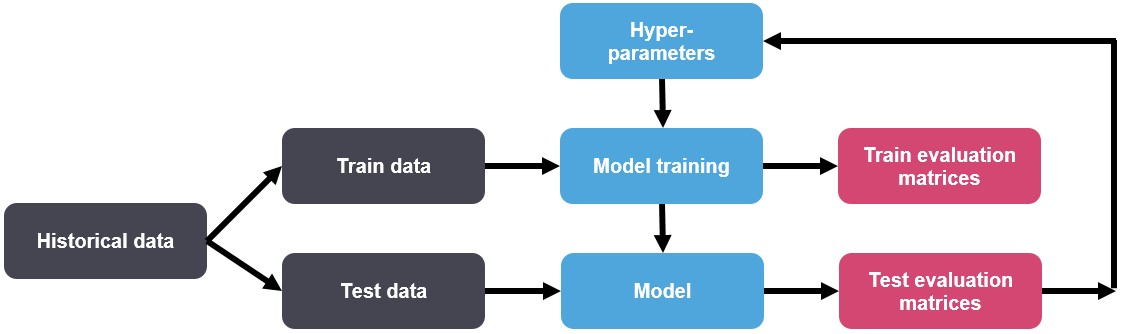# Fine-Tuning Algorithm Parameters in R: A Comprehensive Guide for Effective Machine Learning Models# Fine-Tuning Algorithm Parameters in R: A Comprehensive Guide for Effective Machine Learning Models

## Introduction

Building an effective machine learning model goes beyond just choosing the right algorithm. It involves fine-tuning the algorithm’s parameters for optimal performance. R, a popular language for statistical computing and graphics, provides a range of packages that make parameter tuning more streamlined and effective. This article illuminates the process of algorithm parameter tuning in R.

## Why Algorithm Parameter Tuning Matters

Machine learning algorithms come equipped with parameters that govern their behavior. Some parameters are learned directly from the data (like the coefficients in a linear regression model), while others need to be specified before training. These pre-set parameters, termed hyperparameters, can significantly influence the model’s performance.

Hyperparameters may control aspects like the complexity of a decision tree (e.g., maximum depth) or the penalty term in a support vector machine (C parameter). Choosing the best hyperparameters can significantly improve your model’s predictive accuracy and prevent issues like overfitting and underfitting.

## Hyperparameter Tuning Techniques in R

R provides several packages for hyperparameter tuning, including `caret` and `tuneRanger`. Here’s how you can use these packages for tuning:

### Grid Search with Caret

The caret package in R provides an implementation of grid search, where we specify a set of possible values for each hyperparameter, and the algorithm evaluates the model performance for each combination of these hyperparameters.

Here’s an example of grid search with a support vector machine:

``````library(caret)

data(iris)

# Define the control using a cross-validated grid search
ctrl <- trainControl(method="cv", number=10)

# Define the parameter grid
grid <- expand.grid(C = c(0.1, 1, 10, 100),
sigma = c(0.01, 0.1, 1, 10))

# Perform the grid search
fit <- train(Species ~., data=iris, method="svmRadial",
trControl=ctrl, tuneGrid=grid)
``````

### Random Search with tuneRanger

The `tuneRanger` package in R provides an implementation of random search, which randomly selects combinations of hyperparameters to train the model and find the best set. This can be more efficient than grid search when dealing with many hyperparameters.

Here’s an example using a random forest classifier:

``````library(tuneRanger)

data(iris)

# Prepare the data for training
train_data <- iris[1:100, ]
test_data <- iris[101:150, ]

# Perform the random search
result <- tuneRanger(Species ~., data = train_data,
testdata = test_data, num.trees = 500,
num.threads = 1, iters = 100,
measure = "auc", verbose = FALSE)
``````

Hyperparameter tuning can significantly improve your model’s performance. However, remember that it’s just one part of the broader model building process. The quality of your data, the suitability of the model to your problem, and the effectiveness of your feature engineering all play vital roles.

Hyperparameter tuning is as much an art as a science, and finding the best parameters often involves some trial and error. But with the right tools from R at your disposal, you are well-equipped to create models that deliver the performance you need.

## Relevant Prompts for Further Exploration

1. Explain the concept of hyperparameters in machine learning. How do they differ from model parameters?
2. Discuss the significance of hyperparameter tuning in the context of machine learning.
3. Demonstrate how to perform grid search for hyperparameter tuning in R.
4. Compare and contrast grid search and random search. Discuss the situations where one method may be preferable.
5. What challenges might arise during hyperparameter tuning? How can these be mitigated?
6. Explain how hyperparameter tuning can help prevent overfitting and underfitting.
7. Show how to tune the parameters of a decision tree model in R.
8. Discuss the role of cross-validation in the hyperparameter tuning process.
9. Explore advanced techniques for hyperparameter tuning.
10. Discuss how to define the parameter grid or distribution for hyperparameter tuning in R.
11. Explain how to interpret and evaluate the results of hyperparameter tuning.
12. Show how to tune the parameters of a neural network model in R.
13. Discuss the role of randomness in random search and how it influences the results.
14. Explain how the choice of performance metric can influence the hyperparameter tuning process.
15. Discuss how hyperparameter tuning fits into a broader machine learning workflow in R.

# Personal Career & Learning Guide for Data Analyst, Data Engineer and Data Scientist

A list of FREE programming examples together with eTutorials & eBooks @ SETScholars

# Projects and Coding Recipes, eTutorials and eBooks: The best All-in-One resources for Data Analyst, Data Scientist, Machine Learning Engineer and Software Developer## Find more … …

Machine Learning for Beginners in Python: Fast C Hyperparameter Tuning

The Ultimate Guide to Algorithm Parameter Tuning with Scikit-Learn: Empowering Machine Learning Models

Machine Learning for Beginners in Python: Hyperparameter Tuning Using Random Search